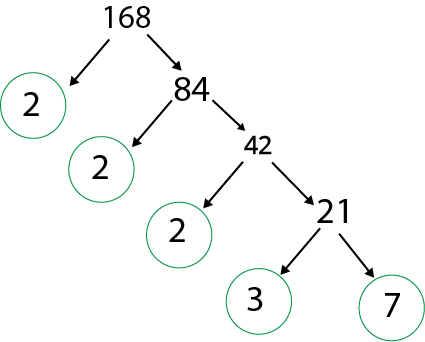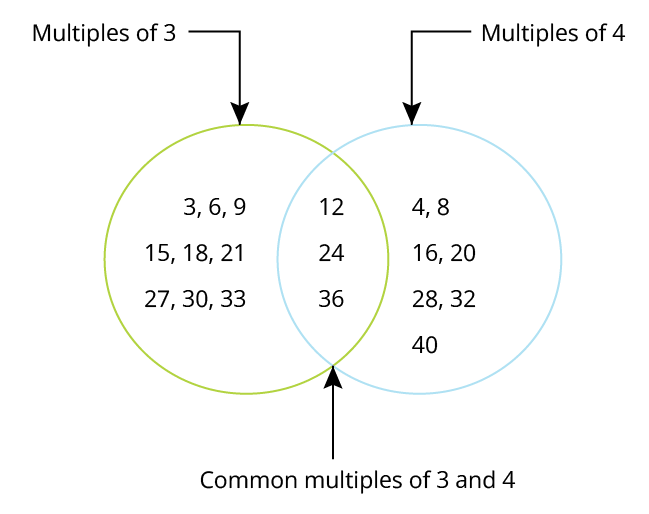Courses
Courses for Kids
Free study material
Offline Centres
More

# Be My Multiple I’ll be your Factor Class 5 Notes CBSE Maths Chapter 6 (Free PDF Download)Last updated date: 29th Nov 2023
Total views: 163.8k
Views today: 1.63k

## NCERT Class 5 Maths Revision Notes and Worksheets: Learn Factors and Multiples

The Class 5 Maths syllabus covers some interesting topics where you will learn the fundamental concepts of factors and multiples. Chapter 6 focuses on teaching how to solve sums by using the knowledge of multiples and factors. The questions are in the form of descriptive word problems and pictorial illustrations. Use the Be My Multiple I'll Be Your Factor revision notes to find out how to extract information from these questions and answer them perfectly.

These revision notes come with worksheets and solutions too. The subject experts at Vedantu have solved the questions using simple multiplication and factorization methods. Solve and practice these worksheets to understand the concepts and applications of multiples and factors.

## Revision Notes Class 5 Mathematics Chapter 6 - Be My Multiple I will be your Factor

### What is a Multiple?

• Multiple is a Product of Number and integer.

• Multiples are often called skip counting or counting by numbers.

• Example: 7 x 8 = 56, from this example we can see that 56 is the multiple of 7.

• Example: Let’s look at another example of multiple:

3 x 1 = 3

3 x 2 = 6

3 x 3 = 9

3 x 4 = 12

Here 3, 6, 9, 12 are the multiples of 3.

Point to Remember - 0 is a multiple of every number as the product of 0 multiplied by any number is 0.

### What is a Factor?

• To get a product, a number is multiplied and these numbers are factors of the product.

• Example: Let us consider the number 6. 6 can be a product of 1 and 6, and 2 and 3. As a result, the factors of 6 are 1, 2, 3, and 6. Therefore, when finding or solving problems related to factors, only positive numbers, whole numbers, and non-fractional numbers are considered.

### Solved Examples

Example 1: What are the Factors of 12?

Ans: The factors 12 are: 1, 2, 3, 4, 6, and 12.

Example 2: What are the factors of 36?

Ans: The number 36 has many factors other than the one and the number itself, thus the factor of 36 are: 1, 2, 3, 4, 6, 9, 12, 18, and 36.

### Factor Tree

• It is a tool used to break down the given number into its prime factors by factorizing the given number.

• We stop this only when we fail to find further factors.

• Example: Now, let us look at the factor tree of 168.Factor tree of 168

Step1: Write the pair factors of the given number which on multiplication results in the same number.

Step2: Mark the prime number as one of the prime factors.

Step3: Repeat step 1 and step 2 on the quotient (ie. the composite number) until you reach the prime number.

Therefore,168 = 2 x 2 x 2 x 3 x 7

### What is a Common Multiple?

• The multiples that are common to two or more numbers are known as the common multiple of those numbers.

• Example: The common multiples of 3 and 4 are:

Multiples of 3: 3, 6, 9, 12, 15, 18, 21, 24, 27, 30, 33, 36.

Multiples of 4: 4, 8, 12, 16, 20, 24, 28, 32, 36, 40.From the multiples of 3 and 4, we can see that 12, 24 and 36 are the common multiple.

Solved Example 1: What are the common multiples of the numbers 10 and 12 by listing multiples?

Ans: Before finding the common multiples of 10 and 12, let us find the multiples of both the number:

Multiples of 10:  10, 20, 30, 40, 50, 60, 70, 80, and 90.

Multiples of 12: 12, 24, 36, 48, 60, 72, 84, 96, and 108.

From the above multiple of 10 and 12, we can see that the least common multiple is 60.

### What are Common Factors?

• A common factor is a factor that is common to two or more numbers.

• Highest common factor: It is the greatest number which divides the two numbers.

• Lowest common factor: It is the smallest number which divides the two numbers.

• Example: To find the biggest or highest common factor of numbers 20 and 40. Let us firstly write down the factors of 20 and 40.

Factors of 20: 1, 2, 5, 4, 10, and 20

Factors of 40: 1, 2, 4, 5, 8, 10, 20, and 40.

From the above, it is pretty clear that 20 is the highest common factor of 20 and 40.

### Practise Questions

Q1. What are the 10 multiples of 5?

Ans : The ten multiples of 5 are: 5, 10, 15, 20, 25, 30, 35, 40, 45, 50.

Q2. What are the smallest common multiples of the numbers 5 and 6?

Ans : The smallest common multiple of 5 and 6 is 30.

Q3. Find out all the factors of 32 from the multiplication chart?

Ans : The factors of 32 are 1, 2, 4, 8, 16, 32, From the table it can be seen that:

• 1 x 32 =  32

• 2 x 16 = 32

• 4 x 8 = 32

## Importance of CBSE Class 5 Maths Chapter 6 Be My Multiple I’ll Be Your Factor

• This chapter introduces you to some important methods used in multiplication and factorization. You will learn how to define the multiples and factors based on the context of questions and formulate solutions.

• Your knowledge of number tables will be used in determining factors and multiples in this chapter. All the questions are framed using stories and pictures. It will keep you engaged and will encourage you to learn the concepts faster.

• You will be able to correlate the tables of single-digit numbers with their factors and multiples. This chapter will explain how identifying common multiples and factors becomes a lot easier with tables.

• Understand the objective of a dice game given in this chapter. Get to know what prime numbers are by rolling dice. Follow the instructions given to find the multiples of different single-digit numbers.

• You will learn how to find out common multiples for given numbers. You will also learn how to identify and circle the bigger number in a given set by seeking information from the questions.

## Benefits of CBSE Class 5 Maths Chapter 6 Worksheets and Revision Notes

• It is easier to understand the meaning and objective of Be My Multiple I'll Be Your Factor summary from the simpler explanation of concepts prepared by our experts.

• Practicing the worksheets and going through the factors and multiples Class 5 solutions help to find out how accurate answers of the given sums.

• You can learn how to solve all the questions following the CBSE guidelines to score better in the exams.

## Download Free Worksheets and Revision Notes for Class 5 Maths Chapter 6

Download the free PDF of revision notes and worksheets for Be My Multiple I will Be Your Factor for your exam preparation. Revise the chapter with the help of these notes and proceed to solve the worksheets. Strengthen your problem-solving and logical reasoning skills with these notes and worksheets.

## Conclusion

"Be My Multiple I'll Be Your Factor" from CBSE Class 5 Maths Chapter 6, encapsulates the fundamental concept of multiplication and factors in an engaging manner. This catchy phrase serves as a mnemonic to help students grasp the relationship between multiples and factors more effectively. The chapter not only imparts mathematical knowledge but also encourages critical thinking and problem-solving skills. With the aid of Vedantu's educational resources, this chapter can be better understood, fostering a strong foundation in mathematics. By embracing these principles, students can navigate the world of numbers with confidence and competence, laying the groundwork for future mathematical success.

## FAQs on Be My Multiple I’ll be your Factor Class 5 Notes CBSE Maths Chapter 6 (Free PDF Download)

1.  Why should I learn to calculate the common factors and multiples?

This chapter builds a conceptual foundation based on factors and multiples. It will be useful to learn LCM, HCF, and GCM in the higher classes.

2.  How can I complete the multiplication chart in this chapter?

Check the left column and the top row. Put your finger on one empty square. Find which column and row connect to it. Multiply the numbers in the specific row and column squares to get your answer.